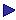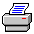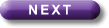Tutorial Contents Tutorial Eight: Relations- Reflexivity - Symmetry -Transitivity - Connectedness - Example of a tableau proof - Eqivalent Relations More Tutorials OneTwoThreeFourFiveSix# 8: Relations

 Binary relations

A binary relation is the sort of relation which can be expressed by a 2-place predicate: for example:

x loves y

x is greater than y

[x is a cow Ù y is a blade of grass]

Hodges uses a convenient way of representing a relation. Consider a relation expressed by "Rxy". Then we represent the domain by a circle, and the individuals in the domain by dots labelled, perhaps, "a", "b", "c", and so on; we represent the fact that Rab, say, by drawing an arrow from dot a to dot b; we represent the fact that both Rab and Rba by drawing a double arrow between a and b; and we represent the fact that Raa by drawing a double arrow looping from a back to itself (a "loop", for short). The whole representation of the relation is its graph.

This way of thinking of relations provides a useful way of describing various properties which a relation may or may not have.Print this page